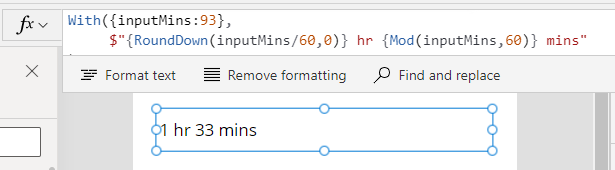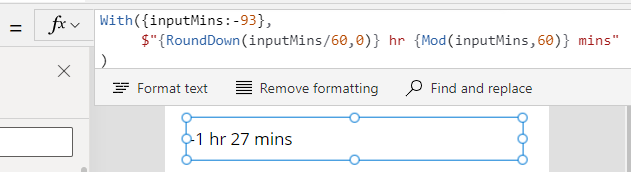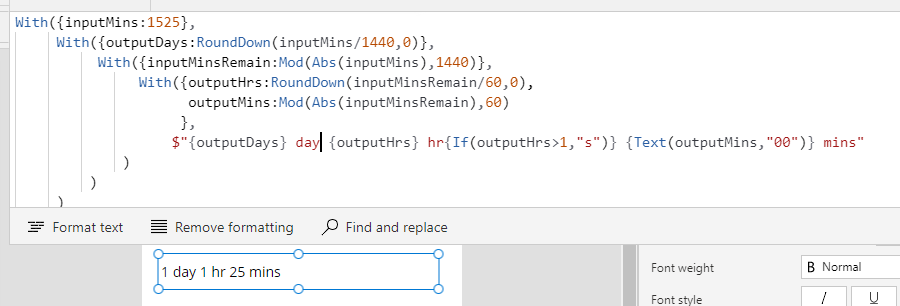# Dates - How to display minutes as hours/minutes, and days/hours/minutes

If you need to convert an input value in minutes into hours and minutes, this post describes the formula to carry out this task.

When working with times, it can be very useful to convert minutes into hours/minutes in order to make the output more readable for the user.

To demonstrate, let's take the example input value of 93 minutes. The basic formula to carry out this task looks like this:

`With({inputMins:93},     \$"{RoundDown(inputMins/60)} hr {Mod(inputMins,60)} mins")`

This produces the target output of "1hr 33mins", as shown belowThe pertinent parts of this formula are the RoundDown and Mod functions. To derive the hour component, we divide the input value by 60 mins and round down the result. To derive the number of minutes, we call the Mod function which returns the remainder when we divide the input value by 60.

An important point about this approach is that it doesn't handle negative input values. Negative values can occur in cases where we determine the input value from a calculation.

To demonstrate, let's take the input value -93mins. The above formula does not return the expected value "1hr 33mins", and instead returns "-1hr 27mins". Because the input value is negative, the Mod function returns the remainder in the opposite direction (27mins).To adapt the formula so that it handles negative numbers, we call Abs on the input value that we supply to the Mod function. This Abs function returns the absolute positive value and ensures that we always work with positive values.

`With({inputMins:-93},     \$"{RoundDown(inputMins/60,0)} hr {Mod(Abs(inputMins),60)} mins")`
As the screenshot below highlights, this now returns the expected result of "-1hr 33mins".Finally, we can further adapt the formula so that it properly handles the pluralisation of the hour label and format the minute component so that it always shows 2 digits. As the example below highlights, an input value of 125 returns "2 hrs 05mins".
.
`With({inputMins:125},           With({outputHrs:RoundDown(inputMins/60,0),            outputMins:Mod(Abs(inputMins),60)           },           \$"{outputHrs} hr{If(outputHrs>1,"s")} {Text(outputMins,"00")} mins"      ))`## Converting minutes to days/hours/minutes

For larger input values, we can further adapt the formula to display the output in days/hours/minutes. We adapt the formula by first dividing and rounding down the input value in mins by 1440 (the number of minutes in a day). This determines the number of days, and we can then implement our earlier syntax to determine the hour and minute components.

`With({inputMins:1525},          With({outputDays:RoundDown(inputMins/1440,0)},          With({inputMinsRemain:Mod(Abs(inputMins),1440)},                    With({outputHrs:RoundDown(inputMinsRemain/60,0),                     outputMins:Mod(Abs(inputMinsRemain),60)                    },                   \$"{outputDays} day                        {outputHrs} hr{If(outputHrs>1,"s")}                        {Text(outputMins,"00")} mins"             )         )     ))`
The screenshot below highlights the result with the example input value of 1525 minutes.## Conclusion

When working with times, it can be very useful to convert minutes into hours/minutes or days/hours/minutes. This post highlighted the formula to carry out this task, including how to account for negative numbers.